Uh Oh! It seems you’re using an Ad blocker!

We always struggled to serve you with the best online calculations, thus, there's a humble request to either disable the AD blocker or go with premium plans to use the AD-Free version for calculators.

Or# Radius of a Circle Calculator

Given:

Circumference (c)

Area (A)

Table of Content

Get the Widget!

Add this calculator to your site and lets users to perform easy calculations.

Feedback

How easy was it to use our calculator? Did you face any problem, tell us!

Our radius of a circle calculator mainly functions to find the radius of a circle from the values of circumference, area, or diameter.

### How Does This Radius of a Circle Calculator Work?

Truly uttering, our calculator is pretty easy to use if you stick to the following usage guide!

Input:

• From the first drop-down list, select the parameter with which you wish to carry the calculations
• After that, enter its value along with the unit selected from the next list
• At last, hit the “Calculate” button

Output:

• Radius, area, diameter, and circumference of the circle

## What is the Radius of a Circle?

You all may be familiar with a circle, a well-known and widely used geometrical figure.

“The distance from the center of a circle to any of its points on the circumference is known as the radius”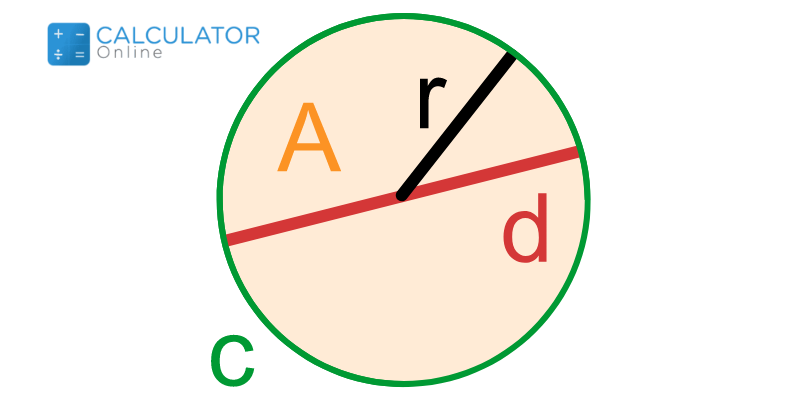## How to Find the Radius of a Circle?

In geometry, a circle is defined by many related entities. And if you are willing to find its radius given different parameters, then these include:

### Radius of a Circle Formula:

#### From Diameter: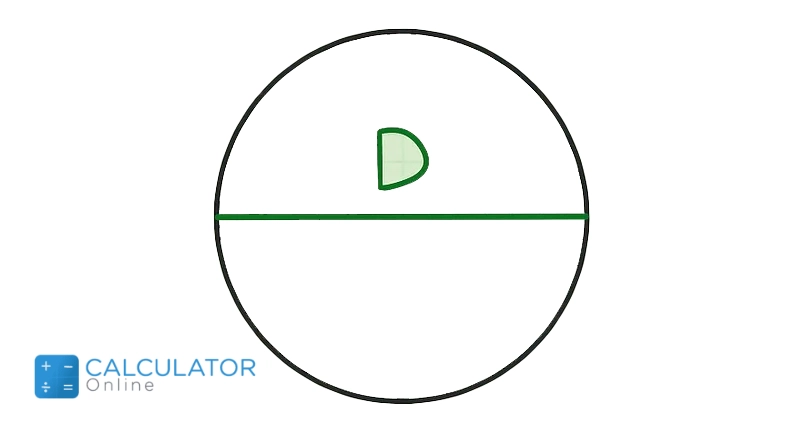As we know that:

$$Diameter=D=2*r$$
or
$$r=\dfrac{D}{2}$$

#### From Area: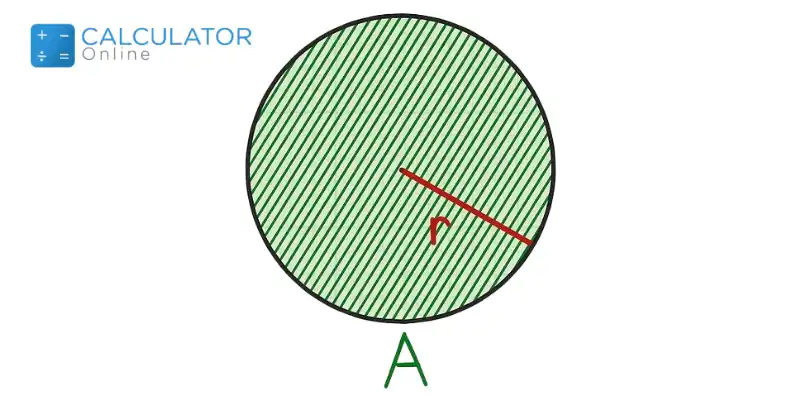You know that:

$$Area=A=𝜋r^{2}$$
or
$$r^{2}=\dfrac{A}{𝜋}) #### From Circumference: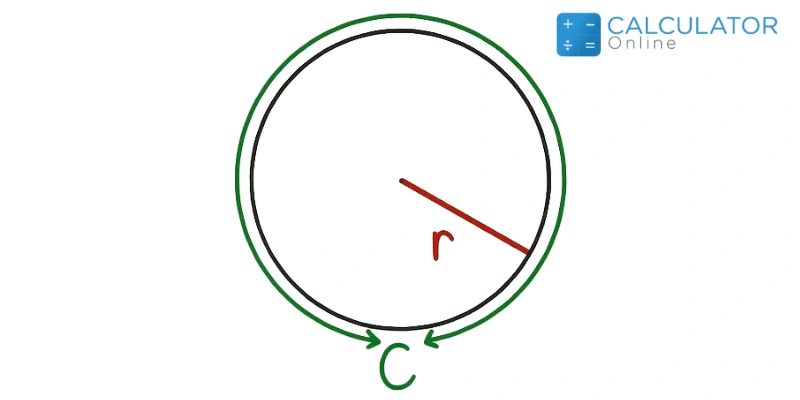As you know that: \(C=2*𝜋*r$$
or
(r=\dfrac{C}{2𝜋}\)

#### From Area and Central Angle of a Sector: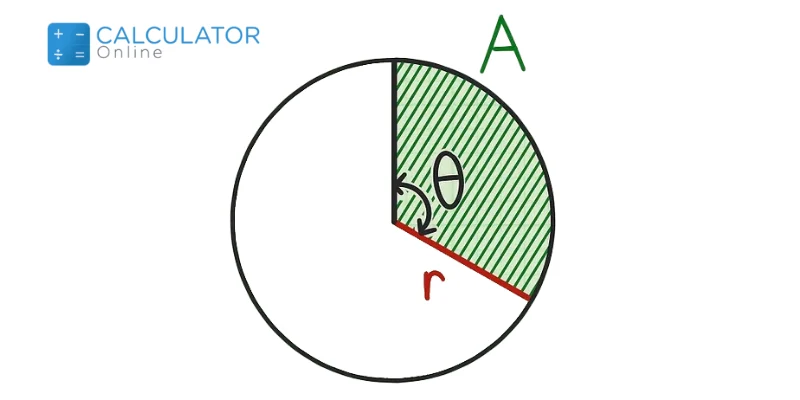You know that:

$$A=\dfrac{\theta}{360^\text{o}}*𝜋*r^{2}$$
or
$$r=sqrt{\dfrac{A*360^\text{o}}{\theta*𝜋}}$$

### Example:

#### Statement:

What’s the radius of a circle having area as $$78m^{2}$$?

##### Solution:

$$r^{2}=\dfrac{A}{𝜋}$$

$$r^{2}=\dfrac{78}{3.14}$$

$$r^{2}=24.840$$

$$r=\sqrt{24.840}$$

$$r=4.983$$

## References:

From the source of Wikipedia: Radius, Formula, Use in coordinate systems

From the source of Khan Academy: Radius, diameter, circumference & π, Labeling, Area of parts of circles

From the source of Lumen Learning: Circles, Equation of a Circle in Standard Form, General form of a circle